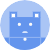search
Search
menu search toc more_vert
Guest 0reps
Thanks for the thanks!
close
account_circle
Profile
exit_to_app
Sign out
help Ask a question
search
keyboard_voice
close
Searching Tips
Search for a recipe:
"Creating a table in MySQL"
Search for an API documentation: "@append"
Search for code: "!dataframe"
Apply a tag filter: "#python"
Useful Shortcuts
/ to open search panel
Esc to close search panel
to navigate between search results
d to clear all current filters
Enter to expand content previewDoc SearchCode Search BetaSORRY NOTHING FOUND!
mic
Start speaking...Voice search is only supported in Safari and Chrome.
Shrink
Navigate to
A
A
brightness_medium
share
arrow_backShareTwitterFacebook
0
thumb_down
0
chat_bubble_outline
0
auto_stories new
settings

# Pandas | crosstab method

Pandas
chevron_right
Documentation
chevron_right
General Functions
schedule Jul 1, 2022
Last updated
local_offer PandasPython
Tags

Pandas `crosstab(~)` method computes the cross tabulation of multiple column variables. By default, the computed statistic is the count. See examples for clarification.

# Parameters

1. `index`link | `array-like`

The values used as the index of the resulting DataFrame.

2. `columns`link | `array-like`

The columns of the resulting DataFrame.

3. `values`link | `array-like` | `optional`

The values used to compute the statistic specified by `agg_func`.

4. `rownames`link | `sequence` | `optional`

The names(s) assigned to the rows in the resulting DataFrame. By default, `rownames=None`.

5. `colnames`link | `sequence` | `optional`

The name(s) assigned to the columns in the resulting DataFrame. By default, `colnames=None`.

6. `aggfunc`link | `function` | `optional`

The function that defined the statistic to compute. The input of this function is `values`, and return value must be the aggregated statistic (e.g. the mean, max and so on).

7. `margins`link | `boolean` | `optional`

Whether to include an additional row and column that indicates the sum of cells row-wise and column-wise. By default, `margins=False`.

8. `margin_names`link | `string` | `optional`

If margins is set to `True`, then `margin_names` is the name of the newly added row and column.

9. `dropna` | `boolean` | `optional`

Whether or not to drop columns that contain just `NaN`. By default, `dropna=False`.

10. `normalize`link | `boolean` or `string` | `optional`

Whether or not to normalize the computed values by dividing by their sum:

Value

Description

Normalize over all values.

`"all"` or `True`

`"index"`

Normalize row-wise.

`"columns"`

Normalize column-wise

`False`

Do not normalize.

By default, `noramlize=False`.

# Return Value

A DataFrame that represents the cross tabulation of multiple factors.

# Examples

Consider the following DataFrame:

``` df = pd.DataFrame({"name":["alex","bob","cathy","doge"], "gender":["male","male","female","male"], "age":[50,20,40,20]})df name gender age0 alex male 501 bob male 202 cathy female 403 doge male 20 ```

## Basic usage

To get the count of the males and females of different ages:

``` pd.crosstab(index=df["age"], columns=df["gender"]) gender female maleage 20 0 240 1 050 0 1 ```

Here, note the following:

• we see a breakdown of the counts of each profile. For instance, we have 2 males who are aged 20.

• the label `gender` is the name assigned to the columns.

## Specifying rownames and colnames

To give specific names to the resulting rows and columns:

``` pd.crosstab(index=df["age"], columns=df["gender"], rownames=["AGE"], colnames=["GENDER"]) GENDER female maleAGE 20 0 240 1 050 0 1 ```

Here, we show the same `df` for your reference:

``` df name gender age0 alex male 501 bob male 202 cathy female 403 doge male 20 ```

## Specifying aggfunc

Instead of the count, we could compute other statistics as well. For instance, to compute the mean age of each gender:

``` pd.crosstab(index=df["gender"], columns=["AGE"], values=df["age"], aggfunc=np.mean) col_0 AGEgender female 40male 30 ```

Notes the following:

• we are using Numpy's `mean(~)` function.

• if you specify `aggfunc`, then `values` must also be specified, and vice versa.

## Specifying margins and margins_name

We can compute the total sum of the statistics row-wise and column-wise by specifying `margins=True`:

``` pd.crosstab(index=df["age"], columns=df["gender"], margins=True) gender female male Allage 20 0 2 240 1 0 150 0 1 1All 1 3 4 ```

You can also rename the newly introduced column and row by setting `margins_name`:

``` pd.crosstab(index=df["age"], columns=df["gender"], margins=True, margins_name="TOTAL") gender female male TOTALage 20 0 2 240 1 0 150 0 1 1TOTAL 1 3 4 ```

## Specifying dropna

Consider the following DataFrame with a missing value:

``` df = pd.DataFrame({"name":["alex","bob","cathy","doge"], "gender":["male","male","female","male"], "age":[30,20,np.NaN,30]})df name gender age0 alex male 30.01 bob male 20.02 cathy female NaN3 doge male 30.0 ```

Computing the cross tabulation using `age` and `gender`:

``` pd.crosstab(index=df["age"], columns=df["gender"]) gender maleage 20.0 130.0 2 ```

Notice how the column for `female`, whose values should be filled with `NaN`, is not there. This is the default behaviour of `dropna=True`.

We can choose to keep the column by setting `dropna=False` like so:

``` pd.crosstab(index=df["age"], columns=df["gender"], dropna=False) gender female maleage 20.0 0 130.0 0 2 ```

Notice how the `NaN` have been filled using `0`s.

## Specifying normalize

Consider the following DataFrame:

``` df = pd.DataFrame({"name":["alex","bob","cathy","doge"], "gender":["male","male","female","male"], "age":[50,20,40,20]})df name gender age0 alex male 501 bob male 202 cathy female 403 doge male 20 ```

By default, `normalize=False`, which means the resulting values are not normalized:

``` pd.crosstab(index=df["age"], columns=df["gender"]) gender female maleage 20 0 240 1 050 0 1 ```

In this context, to normalize is to divide each value by the sum of the values.

### True or "all"

To normalize using all values, simply set `normalize=True` like so:

``` pd.crosstab(index=df["age"], columns=df["gender"], normalize=True) # or normalize="all" gender female maleage 20 0.00 0.5040 0.25 0.0050 0.00 0.25 ```

Here, we get a `0.50` there because `2/(2+1+1)=0.5`.

### "rows"

To normalize row-wise, set `normalize="index"` like so:

``` pd.crosstab(index=df["age"], columns=df["gender"], normalize="index") gender female maleage 20 0.0 1.040 1.0 0.050 0.0 1.0 ```

### "columns"

To normalize column-wise, set `normalize="columns"` like so:

``` pd.crosstab(index=df["age"], columns=df["gender"], normalize="columns") gender female maleage 20 0.0 0.66666740 1.0 0.00000050 0.0 0.333333 ```
mail